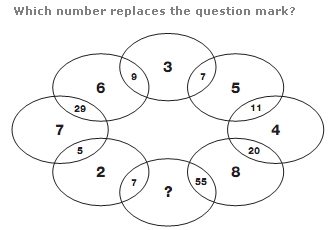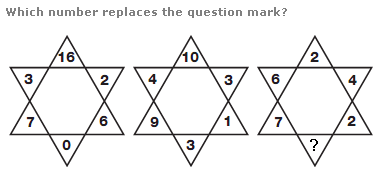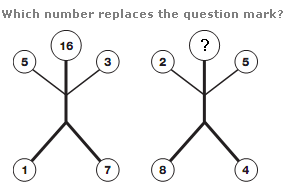# Puzzles - Number puzzles

### Exercise :: Number puzzlesAnswer : 9 Explanation : Multiply numbers in adjacent circles together, then subtract the original numbers, to give the value in the space in-between.Answer : 1 Explanation : In each star, calculate the difference between the 2 left hand and 2 right hand numbers. The value at the top of each star equals the product of these differences, and the value at the bottom of each star equals the difference between these 2 differences.Answer : 6 Explanation : In each figure, calculate the difference between the upper and lower left hand numbers, and the upper and lower right hand numbers and multiply these differences together to give the value at the top.